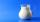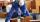# Inlet minus outlet

Water flows into the tank through two pipes and flows out of the third pipe spontaneously. The pipe A itself would fill the tank in 3 hours, the pipe B itself would fille in 4 hours, and the pipe C itself would flow out in 12 hours. How long will the tank be full if all three pipes open at the same time?

Result

t =  2 h

#### Solution:

t*(1/3 + 1/4 - 1/12)=1

6t = 12

t = 2

Calculated by our simple equation calculator.

Leave us a comment of example and its solution (i.e. if it is still somewhat unclear...):

Showing 0 comments:Be the first to comment!#### To solve this example are needed these knowledge from mathematics:

Need help calculate sum, simplify or multiply fractions? Try our fraction calculator. Do you have a linear equation or system of equations and looking for its solution? Or do you have quadratic equation? Tip: Our volume units converter will help you with converion of volume units.

## Next similar examples:

1. Hectoliters of waterThe pool has a total of 126 hectoliters of water. The first pump draws 2.1 liters of water per second. A second pump pumps 3.5 liters of water per second. How long will it take both pumps to drain four-fifths of the water at the same time?
2. Vehicle tankA vehicle tank was 3/5 full of petrol. When 21 liters of fuel was added it was 5/6 full. How many liters of petrol can the tank hold?
3. Cleaning windowsCleaning company has to wash all the windows of the school. The first day washes one-sixth of the windows of the school, the next day three more windows than the first day and the remaining 18 windows washes on the third day. Calculate how many windows ha
4. Unknown numberI think the number - its sixth is 3 smaller than its third.
5. Equation with xSolve the following equation: 2x- (8x + 1) - (x + 2) / 5 = 9
6. Simplify 2Simplify expression: 5ab-7+3ba-9
7. UN 1If we add to an unknown number his quarter, we get 210. Identify unknown number.
8. Equation with fractionsSolve equation: ? It is equation with fractions.
9. EquationSolve the equation: 1/2-2/8 = 1/10; Write the result as a decimal number.
10. Unknown numberIdentify unknown number which 1/5 is 40 greater than one tenth of that number.
11. Simple equationSolve for x: 3(x + 2) = x - 18
12. MilkAt the kindergarten, every child got 1/5 liter of milk in the morning and another 1/8 liter of milk in the afternoon. How many liters were consumed per day for 20 children?
13. PearsThere were pears in the basket, I took two-fifths of them, and left six in the basket. How many pears did I take?
14. Bricklayers8 bricklayers build a house for 630 days. How many bricklayers have to add after 150 days, so then the whole building completed in (next) 320 days?
15. Boys and girlsThere are 28 girls in the hall. 5/7 of all children are boys. How many children and how many boys are there?
16. TogetherGrandfather, father, and son are carpenters. They decided to make a wardrobe. Father produces it for 12 hours, his son for 15 hours, and grandfather for 10 hours. How long will they make it together?
17. EqnSolve equation with fractions: 2x/3-50=40+x/4